# Image Caption Generator using Deep Learning on Flickr8K dataset

Generating a caption for a given image is a challenging problem in the deep learning domain. In this article, we will use different techniques of computer vision and NLP to recognize the context of an image and describe them in a natural language like English. we will build a working model of the image caption generator by using CNN (Convolutional Neural Networks) and LSTM (Long short term memory) units.
For training our model I’m using Flickr8K dataset. It consists of 8000 unique images and each image will be mapped to five different sentences which will describe the image.

Step 1: Import the required libraries

 `# linear algebra ` `import` `numpy as np   ` `# data processing, CSV file I / O (e.g. pd.read_csv) ` `import` `pandas as pd   ` `import` `os ` `import` `tensorflow as tf ` `from` `keras.preprocessing.sequence ``import` `pad_sequences ` `from` `keras.preprocessing.text ``import` `Tokenizer ` `from` `keras.models ``import` `Model ` `from` `keras.layers ``import` `Flatten, Dense, LSTM, Dropout, Embedding, Activation ` `from` `keras.layers ``import` `concatenate, BatchNormalization, ``Input` `from` `keras.layers.merge ``import` `add ` `from` `keras.utils ``import` `to_categorical, plot_model ` `from` `keras.applications.inception_v3 ``import` `InceptionV3, preprocess_input ` `import` `matplotlib.pyplot as plt  ``# for plotting data ` `import` `cv2 `

The format of our file is image and caption separated by a newline (“\n”) i.e, it consists of the name of the image followed by a space and the description of the image in CSV format. Here we need to map the image to its descriptions by storing them in a dictionary.

 `def` `load_description(text): ` `    ``mapping ``=` `dict``() ` `    ``for` `line ``in` `text.split(``"\n"``): ` `        ``token ``=` `line.split(``"\t"``) ` `        ``if` `len``(line) < ``2``:   ``# remove short descriptions ` `            ``continue` `        ``img_id ``=` `token[``0``].split(``'.'``)[``0``] ``# name of the image ` `        ``img_des ``=` `token[``1``]              ``# description of the image ` `        ``if` `img_id ``not` `in` `mapping: ` `            ``mapping[img_id] ``=` `list``() ` `        ``mapping[img_id].append(img_des) ` `    ``return` `mapping ` ` `  `token_path ``=` `'/kaggle / input / flickr8k / flickr_data / Flickr_Data / Flickr_TextData / Flickr8k.token.txt'` `text ``=` `open``(token_path, ``'r'``, encoding ``=` `'utf-8'``).read() ` `descriptions ``=` `load_description(text) ` `print``(descriptions[``'1000268201_693b08cb0e'``])`

Output:

```['A child in a pink dress is climbing up a set of stairs in an entry way .',

'A girl going into a wooden building .',

'A little girl climbing into a wooden playhouse .',

'A little girl climbing the stairs to her playhouse .',

'A little girl in a pink dress going into a wooden cabin .']
```

Step 3: Cleaning the text
One of the main steps in NLP is to remove noise so that the machine can detect the patterns easily in the text. Noise will be present in the form of special characters such as hashtags, punctuation and numbers. All of which are difficult for computers to understand if they are present in the text. So we need to remove these for better results. Additionally, you can also remove stop words and perform Stemming and Lemmatization by using NLTK library.

 `def` `clean_description(desc): ` `    ``for` `key, des_list ``in` `desc.items(): ` `        ``for` `i ``in` `range``(``len``(des_list)): ` `            ``caption ``=` `des_list[i] ` `            ``caption ``=` `[ch ``for` `ch ``in` `caption ``if` `ch ``not` `in` `string.punctuation] ` `            ``caption ``=` `''.join(caption) ` `            ``caption ``=` `caption.split(``' '``) ` `            ``caption ``=` `[word.lower() ``for` `word ``in` `caption ``if` `len``(word)>``1` `and` `word.isalpha()] ` `            ``caption ``=` `' '``.join(caption) ` `            ``des_list[i] ``=` `caption ` ` `  `clean_description(descriptions) ` `descriptions[``'1000268201_693b08cb0e'``]`

Step 4: Generate the Vocabulary

Vocabulary is a set of unique words which are present in our text corpus. When processing raw text for NLP, everything is done around the vocabulary.

 `def` `to_vocab(desc): ` `    ``words ``=` `set``() ` `    ``for` `key ``in` `desc.keys(): ` `        ``for` `line ``in` `desc[key]: ` `            ``words.update(line.split()) ` `    ``return` `words ` `vocab ``=` `to_vocab(descriptions)`

Here we need to map the images in the training set to their corresponding descriptions which are present in our descriptions variable. Create a list of names of all training images and then create an empty dictionary and map the images to their descriptions using image name as key and a list of descriptions as its value. while mapping the descriptions add unique words at the beginning and end to identify the start and end of the sentence.

 `import` `glob ` `images ``=` `'/kaggle / input / flickr8k / flickr_data / Flickr_Data / Images/'` `# Create a list of all image names in the directory ` `img ``=` `glob.glob(images ``+` `'*.jpg'``) ` ` `  `train_path ``=` `'/kaggle / input / flickr8k / flickr_data / Flickr_Data / Flickr_TextData / Flickr_8k.trainImages.txt'` `train_images ``=` `open``(train_path, ``'r'``, encoding ``=` `'utf-8'``).read().split(``"\n"``) ` `train_img ``=` `[]  ``# list of all images in training set ` `for` `im ``in` `img: ` `    ``if``(im[``len``(images):] ``in` `train_images): ` `        ``train_img.append(im) ` `         `  `# load descriptions of training set in a dictionary. Name of the image will act as ey ` `def` `load_clean_descriptions(des, dataset): ` `    ``dataset_des ``=` `dict``() ` `    ``for` `key, des_list ``in` `des.items(): ` `        ``if` `key``+``'.jpg'` `in` `dataset: ` `            ``if` `key ``not` `in` `dataset_des: ` `                ``dataset_des[key] ``=` `list``() ` `            ``for` `line ``in` `des_list: ` `                ``desc ``=` `'startseq '` `+` `line ``+` `' endseq'` `                ``dataset_des[key].append(desc) ` `    ``return` `dataset_des ` ` `  `train_descriptions ``=` `load_clean_descriptions(descriptions, train_images) ` `print``(train_descriptions[``'1000268201_693b08cb0e'``])`

Output:

```['startseq child in pink dress is climbing up set of stairs in an entry way endseq',

'startseq girl going into wooden building endseq',

'startseq little girl climbing into wooden playhouse endseq',

'startseq little girl climbing the stairs to her playhouse endseq',

'startseq little girl in pink dress going into wooden cabin endseq']
```

Step 6: Extract the feature vector from all images

Now we will give an image as an input to our model but unlike humans, machines cannot understand the image by seeing them. So we need to convert the image into an encoding so that the machine can understand the patterns in it. For this task, I’m using transfer learning i.e, we use a pre-trained model that has been already trained on large datasets and extract the features from these models and use them for our work. Here I’m using the InceptionV3 model which has been trained on Imagenet dataset that had 1000 different classes to classify. We can directly import this model from Keras.applications module.

We need to remove the last classification layer to get the (2048, ) dimensional feature vector from InceptionV3 model.

 `from` `keras.preprocessing.image ``import` `load_img, img_to_array ` `def` `preprocess_img(img_path): ` `    ``# inception v3 excepts img in 299 * 299 * 3 ` `    ``img ``=` `load_img(img_path, target_size ``=` `(``299``, ``299``)) ` `    ``x ``=` `img_to_array(img) ` `    ``# Add one more dimension ` `    ``x ``=` `np.expand_dims(x, axis ``=` `0``) ` `    ``x ``=` `preprocess_input(x) ` `    ``return` `x ` ` `  `def` `encode(image): ` `    ``image ``=` `preprocess_img(image) ` `    ``vec ``=` `model.predict(image) ` `    ``vec ``=` `np.reshape(vec, (vec.shape[``1``])) ` `    ``return` `vec ` ` `  `base_model ``=` `InceptionV3(weights ``=` `'imagenet'``) ` `model ``=` `Model(base_model.``input``, base_model.layers[``-``2``].output) ` `# run the encode function on all train images and store the feature vectors in a list ` `encoding_train ``=` `{} ` `for` `img ``in` `train_img: ` `    ``encoding_train[img[``len``(images):]] ``=` `encode(img) `

Step 7: Tokenizing the vocabulary

In this step, we need to tokenize all the words present in our vocabulary. Alternatively, we can use tokenizer in Keras to do this task.

 `# list of all training captions ` `all_train_captions ``=` `[] ` `for` `key, val ``in` `train_descriptions.items(): ` `    ``for` `caption ``in` `val: ` `        ``all_train_captions.append(caption) ` ` `  `# consider only words which occur atleast 10 times ` `vocabulary ``=` `vocab ` `threshold ``=` `10` `# you can change this value according to your need ` `word_counts ``=` `{} ` `for` `cap ``in` `all_train_captions: ` `    ``for` `word ``in` `cap.split(``' '``): ` `        ``word_counts[word] ``=` `word_counts.get(word, ``0``) ``+` `1` ` `  `vocab ``=` `[word ``for` `word ``in` `word_counts ``if` `word_counts[word] >``=` `threshold] ` ` `  `# word mapping to integers ` `ixtoword ``=` `{} ` `wordtoix ``=` `{} ` ` `  `ix ``=` `1` `for` `word ``in` `vocab: ` `    ``wordtoix[word] ``=` `ix ` `    ``ixtoword[ix] ``=` `word ` `    ``ix ``+``=` `1` `     `  `# find the maximum length of a description in a dataset ` `max_length ``=` `max``(``len``(des.split()) ``for` `des ``in` `all_train_captions) ` `max_length`

Step 8: Glove vector embeddings

GloVe stands for global vectors for word representation. It is an unsupervised learning algorithm developed by Stanford for generating word embeddings by aggregating global word-word co-occurrence matrix from a corpus. Also, we have 8000 images and each image has 5 captions associated with it. It means we have 30000 examples for training our model. As there are more examples you can also use data generator for feeding input in the form of batches to our model rather than giving all at one time. For simplicity, I’m not using this here.

Also, we are going to use an embedding matrix to store the relations between words in our vocabulary. An embedding matrix is a linear mapping of the original space to a real-valued space where entities will have meaningful relationships.

 `X1, X2, y ``=` `list``(), ``list``(), ``list``() ` `for` `key, des_list ``in` `train_descriptions.items(): ` `    ``pic ``=` `train_features[key ``+` `'.jpg'``] ` `    ``for` `cap ``in` `des_list: ` `        ``seq ``=` `[wordtoix[word] ``for` `word ``in` `cap.split(``' '``) ``if` `word ``in` `wordtoix] ` `        ``for` `i ``in` `range``(``1``, ``len``(seq)): ` `            ``in_seq, out_seq ``=` `seq[:i], seq[i] ` `            ``in_seq ``=` `pad_sequences([in_seq], maxlen ``=` `max_length)[``0``] ` `            ``out_seq ``=` `to_categorical([out_seq], num_classes ``=` `vocab_size)[``0``] ` `            ``# store ` `            ``X1.append(pic) ` `            ``X2.append(in_seq) ` `            ``y.append(out_seq) ` ` `  `X2 ``=` `np.array(X2) ` `X1 ``=` `np.array(X1) ` `y ``=` `np.array(y) ` ` `  `# load glove vectors for embedding layer ` `embeddings_index ``=` `{} ` `golve_path ``=``'/kaggle / input / glove-global-vectors-for-word-representation / glove.6B.200d.txt'` `glove ``=` `open``(golve_path, ``'r'``, encoding ``=` `'utf-8'``).read() ` `for` `line ``in` `glove.split(``"\n"``): ` `    ``values ``=` `line.split(``" "``) ` `    ``word ``=` `values[``0``] ` `    ``indices ``=` `np.asarray(values[``1``: ], dtype ``=` `'float32'``) ` `    ``embeddings_index[word] ``=` `indices ` ` `  `emb_dim ``=` `200` `emb_matrix ``=` `np.zeros((vocab_size, emb_dim)) ` `for` `word, i ``in` `wordtoix.items(): ` `    ``emb_vec ``=` `embeddings_index.get(word) ` `    ``if` `emb_vec ``is` `not` `None``: ` `        ``emb_matrix[i] ``=` `emb_vec ` `emb_matrix.shape`

Step 9: Define the model
For defining the structure of our model, we will be using the Keras Model from Functional API. It has three major steps:

• Processing the sequence from the text
• Extracting the feature vector from the image
• Decoding the output by concatenating the above two layers

 `# define the model ` `ip1 ``=` `Input``(shape ``=` `(``2048``, )) ` `fe1 ``=` `Dropout(``0.2``)(ip1) ` `fe2 ``=` `Dense(``256``, activation ``=` `'relu'``)(fe1) ` `ip2 ``=` `Input``(shape ``=` `(max_length, )) ` `se1 ``=` `Embedding(vocab_size, emb_dim, mask_zero ``=` `True``)(ip2) ` `se2 ``=` `Dropout(``0.2``)(se1) ` `se3 ``=` `LSTM(``256``)(se2) ` `decoder1 ``=` `add([fe2, se3]) ` `decoder2 ``=` `Dense(``256``, activation ``=` `'relu'``)(decoder1) ` `outputs ``=` `Dense(vocab_size, activation ``=` `'softmax'``)(decoder2) ` `model ``=` `Model(inputs ``=` `[ip1, ip2], outputs ``=` `outputs)`

Output: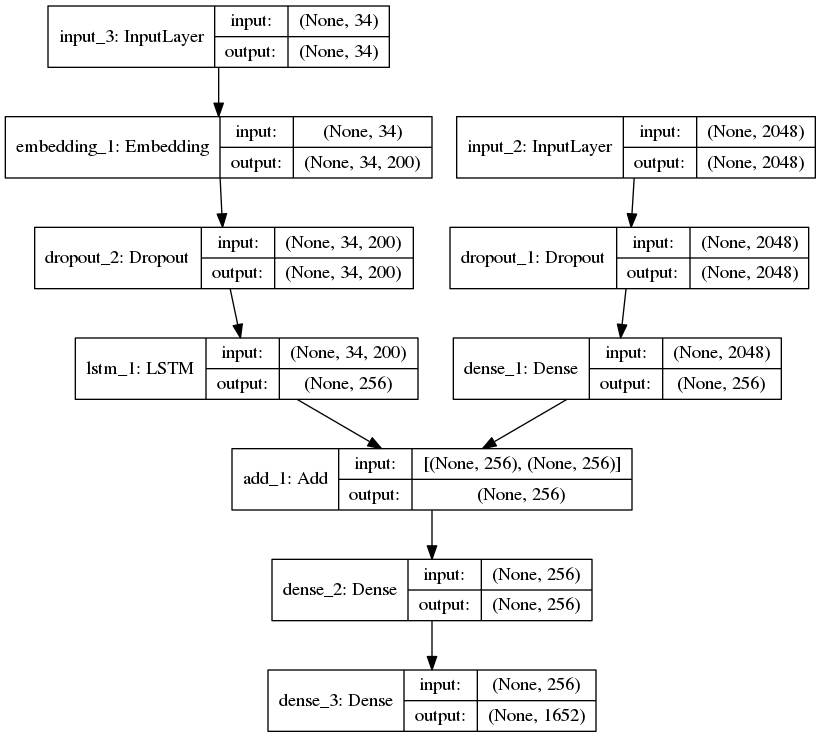Caption Generator deep learning model

Step 10: Training the model

For training our model I’m using Adam’s optimizer and loss function as categorical cross-entropy. I’m training the model for 50 epochs which will be enough for predicting the output. In case you have more computational power (no. of GPU’s) you can train it by decreasing batch size and increasing number of epochs.

 `model.layers[``2``].set_weights([emb_matrix]) ` `model.layers[``2``].trainable ``=` `False` `model.``compile``(loss ``=` `'categorical_crossentropy'``, optimizer ``=` `'adam'``) ` `model.fit([X1, X2], y, epochs ``=` `50``, batch_size ``=` `256``) ` `# you can increase the number of epochs for better results`

Output:

```Epoch 1/1

292328/292328 [==============================] - 55s 189us/step - loss: 3.8895

Epoch 1/1

292328/292328 [==============================] - 55s 187us/step - loss: 3.1549

Epoch 1/1

292328/292328 [==============================] - 54s 186us/step - loss: 2.9185

Epoch 1/1

292328/292328 [==============================] - 54s 186us/step - loss: 2.7652

Epoch 1/1

292328/292328 [=================>.........] - ETA: 15s - loss: 2.6496
```

Step 11: Predicting the output

 `def` `greedy_search(pic): ` `    ``start ``=` `'startseq'` `    ``for` `i ``in` `range``(max_length): ` `        ``seq ``=` `[wordtoix[word] ``for` `word ``in` `start.split() ``if` `word ``in` `wordtoix] ` `        ``seq ``=` `pad_sequences([seq], maxlen ``=` `max_length) ` `        ``yhat ``=` `model.predict([pic, seq]) ` `        ``yhat ``=` `np.argmax(yhat) ` `        ``word ``=` `ixtoword[yhat] ` `        ``start ``+``=` `' '` `+` `word ` `        ``if` `word ``=``=` `'endseq'``: ` `            ``break` `    ``final ``=` `start.split() ` `    ``final ``=` `final[``1``:``-``1``] ` `    ``final ``=` `' '``.join(final) ` `    ``return` `final`

OUTPUT: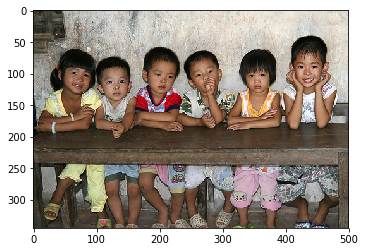Predicted Output: four girls are sitting on wooden floor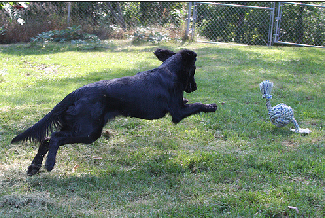Predicted Output: black dog is running through the grass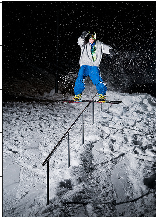Predicted Output: man is skateboarding on ramp

Attention geek! Strengthen your foundations with the Python Programming Foundation Course and learn the basics.

To begin with, your interview preparations Enhance your Data Structures concepts with the Python DS Course.

My Personal Notes arrow_drop_up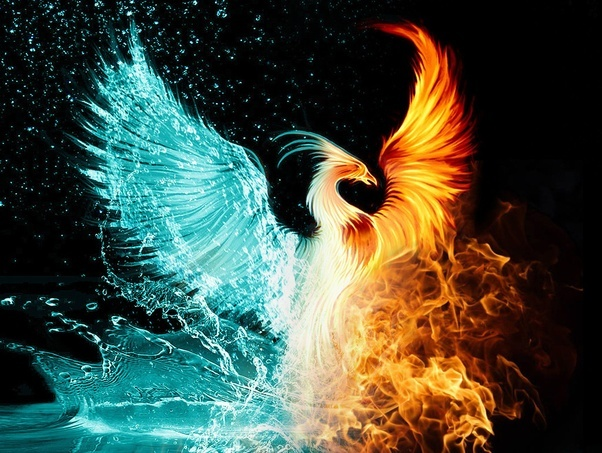Check out this Author's contributed articles.

If you like GeeksforGeeks and would like to contribute, you can also write an article using contribute.geeksforgeeks.org or mail your article to contribute@geeksforgeeks.org. See your article appearing on the GeeksforGeeks main page and help other Geeks.

Please Improve this article if you find anything incorrect by clicking on the "Improve Article" button below.

Article Tags :
Practice Tags :

1

Please write to us at contribute@geeksforgeeks.org to report any issue with the above content.